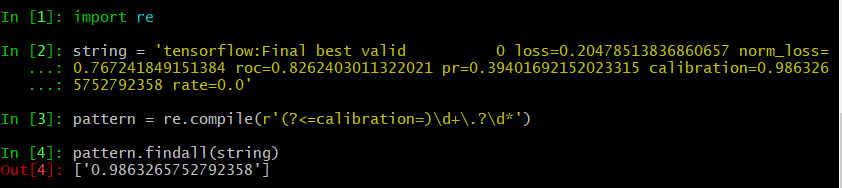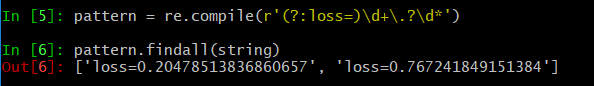• 从string中提取数字
2021-11-13 20:42:51

1、下列程序运行时，输入为：stand*3#count5of6!  （换行回车符），请分析程序，写出运行结果。

#include <stdio.h>
void main()
{
char *str,string;
int number,i;
i=0,number=0;
gets(string);
str=string;
for (i=0; *(str+i)!='\0'; i++)
if (*(str+i)>='0'&&*(str+i)<='9')
number=number*10+*(str+i)-'0';
puts(str);
printf("number=%d\n",number);
}


要注意的点是，指针指向的是字符数组，*（str+i）指向的是单个字符，所以将其进行比较要用'0'和'9',但是其本质是ASCII码的比较所以按下面这样改为数字也可以

#include <stdio.h>
int main()
{
char *str,string;
int number,i;
i=0,number=0;
gets(string);
str=string;
for (i=0; *(str+i)!='\0'; i++)
if (*(str+i)>=48&&*(str+i)<=57)字符串 数组 ascii码表
更多相关内容
•java
• 先使用trim()方法去掉Sting所有起始和结尾... * String 中提取数字 * */ public static String getNumberFromString(String string){ String str = string; //"I23love234you3423java"; str = str.trim(); .
先使用trim()方法去掉Sting中所有起始和结尾的空格，然后获取一个个的char字符，根据ASCII码的值判断是否是数字。如下方法

    /**
* 从 String 中提取数字
* */
public static String getNumberFromString(String string){
String str = string; //"I23love234you3423java";
str = str.trim();
StringBuffer str2 = new StringBuffer();  // 此处可以也使用StringBuffer
if(str != null && !"".equals(str)) {
for (int i = 0; i < str.length(); i++) {
if (str.charAt(i) >= 48 && str.charAt(i) <= 57) {
String s = String.valueOf(str.charAt(i));
// 为什么不用＋号拼接，当然是因为＋号拼接效率最慢的问题啊
str2.append(s);
}
}
}
return str2.toString();
}

上述方法中为什么不用＋号拼接字符串，是因为＋号拼接效率最慢啊。除了方法中使用的StringBuffer，也可以使用StringBuilder，效率很快。

详细的可以看一下这篇博客：Java 如何从String字符串中提取数字2_古时明月2333的博客-CSDN博客

展开全文java
• 直接上代码：float to_float(string s) { int i = 0,n = 0; int point_index = s.find('.'); float result = 0,under_0 = 0;//under_0存储小数部分 if (count(s.begin(), s.end(), '.') > 1) {

直接上代码：

#include <iostream>
#include <string>

using namespace std;

float to_float(string s)
{
int i = 0,n = 0;
int point_index = s.find('.');
float result = 0,under_0 = 0;//under_0存储小数部分
if (count(s.begin(), s.end(), '.') > 1)
{
return 0;//字符串里只能有1个或0个小数点，不然出错退出
}
if (s.find('.') != -1)//字符串里有小数点
{
if ((point_index == 0) || (point_index == s.size()-1))//小数点位置合理，不能在字符串第1位,且不能在最后一位
{
return 0;
}
for (i = 0; i <= point_index - 1; i++)//计算整数部分
{
if (s[i] >= '0'&&s[i] <= '9')
{
result = result * 10 + s[i] - 48;
}
}
for (i = s.size() - 1; i >= point_index-1 ; i--)//计算小数部分
{
if (s[i] >= '0'&&s[i] <= '9')
{
if (i == point_index-1)
{
under_0 = under_0 * 0.1 + 0;//i=小数点前一位，under_0+0
}
else
{
under_0 = under_0* 0.1 + s[i] - 48;
}
}
}
result = result + under_0;//把整数部分和小数部分相加
}
else//字符串只有整数部分
{
for (i = 0; i <= s.size() - 1;i++)
{
if (s[i] >= '0'&&s[i] <= '9')
{
result= result * 10 + s[i] - 48;
}
}
}

return result;
}  
展开全文• python字符串中提取数字 使用正则表达式，用法如下： ## 总结 ## ^ 匹配字符串的开始。 ## $匹配字符串的结尾。 ## \b 匹配一个单词的边界。 ## \d 匹配任意数字。 ## \D 匹配任意非数字字符。 ## x? 匹配一个... # python从字符串中提取数字 ###### 使用正则表达式，用法如下： ## 总结 ## ^ 匹配字符串的开始。 ##$ 匹配字符串的结尾。
## \b 匹配一个单词的边界。
## \d 匹配任意数字。
## \D 匹配任意非数字字符。
## x? 匹配一个可选的 x 字符 (换言之，它匹配 1 次或者 0 次 x 字符)。
## x* 匹配0次或者多次 x 字符。
## x+ 匹配1次或者多次 x 字符。
## x{n,m} 匹配 x 字符，至少 n 次，至多 m 次。
## (a|b|c) 要么匹配 a，要么匹配 b，要么匹配 c。
## (x) 一般情况下表示一个记忆组 (remembered group)。你可以利用 re.search 函数返回对象的 groups() 函数获取它的值。
## 正则表达式中的点号通常意味着 “匹配任意单字符”

###### 解题思路：
1. 既然是提取数字，那么数字的形式一般是：整数，小数，整数加小数；
2. 所以一般是形如：----.-----
3. 根据上述正则表达式的含义，可写出如下的表达式："\d+\.?\d*"；
4. \d+匹配1次或者多次数字，注意这里不要写成*，因为即便是小数，小数点之前也得有一个数字；\.?这个是匹配小数点的，可能有，也可能没有；\d*这个是匹配小数点之后的数字的，所以是0个或者多个；
###### 代码如下：
import re


string="A1.45，b5，6.45，8.82"
print re.findall(r"\d+.?\d*",string)

# 匹配指定字符串开头的数字

例如下面的string：

tensorflow:Final best valid 0 loss=0.20478513836860657 norm_loss=0.767241849151384 roc=0.8262403011322021 pr=0.39401692152023315 calibration=0.9863265752792358 rate=0.0

提取 calibration=0.9863265752792358 .

# 匹配“calibration=”后面的数字
pattern = re.compile(r'(?<=calibration=)\d+\.?\d*')
pattern.findall(string)


# ['0.9863265752792358']# 匹配包含指定字符串开头的数字

pattern = re.compile(r'(?:loss=)\d+\.?\d*')
pattern.findall(string)


# ['loss=0.20478513836860657', 'loss=0.767241849151384']# 匹配时间，17:35:24

string = "WARNING:tensorflow: 20181011 15:28:39 Initialize training"
pattern = re.compile(r'\d{2}:\d{2}:\d{2}')
pattern.findall(string)


# ['15:28:39']

## 匹配时间，20181011 15:28:39

string = "WARNING:tensorflow: 20181011 15:28:39 Initialize training"
pattern = re.compile(r'\d{4}\d{2}\d{2}\s\d{2}:\d{2}:\d{2}')
pattern.findall(string)


# ['20181011 15:28:39']

展开全文python 正则表达式
• 字符串取出数字 第一种 public class Text { public static void main(String[] args) { // 定义一个字符串 String str = "ab12cd34sd56"; // 用java的内部类 str = str.trim(); String str2 = "";...
• ## python 从字符串中提取数字

万次阅读 多人点赞 2021-05-25 23:05:50
方法：re.findall() 使用正则表达式，直接上代码！！！ # 导入正则表达式模块 import re ...# 含有数字的字符串（可以看到有小数和整数） string = "#$1.23，zimu3，520.1314,...数字输出结果：['1', '23', '3', '520',python 正则表达式 字符串 编程语言 • python字符串中提取数字 使用正则表达式，用法如下： ## 总结 ## ^ 匹配字符串的开始。 ##$ 匹配字符串的结尾。 ## \b 匹配一个单词的边界。 ## \d 匹配任意数字。 ## \D 匹配任意非数字字符。 ## x? 匹配一个可...
• public static void main(String[] args) { String a = "adsasdas32a12"; Main main = new Main(); int digitStr = isDigitStr(a); System.out.println(digitStr); } public static int isDigitStr(Stringjava
• 展开全部使用正62616964757a686964616fe58685e5aeb931333431373239则...## \d 匹配任意数字。## \D 匹配任意非数字字符。## x? 匹配一个可选的 x 字符 (换言之，它匹配 1 次或者 0 次 x 字符)。## x* 匹配0次或者多...
• 实例如下： ...//从string中提取数字简单的方法，调用stringstream #include #include #include #include using namespace std; int main( ) { int a; stringstream ss; // ss " apples in my car
• 我将提取字符串包含的所有数字。 哪个更适合于目的，正则表达式或isdigit()方法？例：line = "hello 12 hi 89"结果：[12, 89]#1楼@jmnas，我很喜欢您的回答，但没有找到浮点数。 我正在处理一个脚本，以分析要送入...
• 图1可以使用数组公式：=1*MID(A1,MATCH(TRUE,ISNUMBER(1*MID(A1,ROW($1:$20),1)),0),COUNT(1*MID(A1,...图2公式，使用MID(A1,ROW($1:$20),1)分解单元格A1的文本，使用MATCH(TRUE,ISNUMBER(1*MID(A1,ROW($1:$20),...
• String A = "12", B = "7" A.compareTo(B); 当A大于B时，返回值是一个正整数，即大于0； A.compareTo(B) > 0; 当A等于B时，返回值是0； A.compareTo(B) == 0; 当A小于B时，返回值是一个负整数，即小于0； A....字符串 java 数据结构
• 那我们如何把其中的数字提取出来呢？大致有以下几种方法，正则表达式，集合类，还有就是String类提供的方法。1String类提供的方法:package 测试练习;import java.util.*;public class get_StringNum {/***2012.6...
• JAVA：String str = "asa12dsds546fdf789"; public static void main(String[] args) { int i = 1; String str = "asa12dsds546fdf789"; Pattern pattern = Pattern....
• 那我们如何把其中的数字提取出来呢？大致有以下几种方法，正则表达式，集合类，还有就是string类提供的方法。1 string类提供的方法:package 测试练习;import java.util.*;public class get_stringnum {/***2016.1...
• /// 字符串获取数字（） /// </summary> /// <param name="str"></param> /// <returns></returns> public static decimal GetNumberInStr(string str) ...
• 逛到一个有意思的博客在里面看到一篇关于ValueError: invalid literal for int() with base ...在Python3.5.2 官方文档re模块sub函数的定义是：  re.sub(pattern, repl, string, count=0, flags=0) 在字符串 string
• 方法一：parseInt()方法 针对前面是数字,后面非数字的情况 例： var string ="123测试"；...使用正则表达式将字符串的非字符删除掉就行: 例： var string ="123测试"； var num1=string.replace(/[^0-正则表达式
• 那我们如何把其中的数字提取出来呢？大致有以下几种方法，正则表达式，集合类，还有就是String类提供的方法。 1 String类提供的方法: package 测试练习; import java.util.*; public class get_StringNum
• #include <qregexp.h> #include <qdebug.h> QString a = "32A"; QRegExp rx("\\d+"); QString d; int data_d; rx.indexIn(a,0); d = rx.cap(0); data_d = d.toInt(); qDebug()<...正则表达式
• 习22.SQL11.的一些函数' --patindex函数返回所查内容在字符串第一次出现的内容print patindex('%在%',@Name) --此处会返回3 print patindex('%[0-9]%',@Name) --此处会返回4 --STUFF函数删除指定长度的字符在...
• 如果字符串包含数字如日期，并转换日期 String a="2019年11月23日15：10时"; String regEx="[^0-9]"; Pattern p = Pattern.compile(regEx); Matcher m = p.matcher(a); String time = m.replaceAll("").trim()....Java 正则表达式
• I have a requirement to find and extract a number contained within a string. 我需要查找并提取字符串包含的数字。 Fregex
• //如string=“TAI-4564878”，获取数字 public class getDataFromString { public static String getData(String str) { //String a=“love23next234csdn3423javaeye”; String regEx="[^0-9]"; Pattern p = ...
• I have a list of strings that I am trying to parse for data that is meaningful to ... I need an ID number that is contained within the string. Sometimes it might be two or even three of them. Example ...
• gfg 就是你要处理的含有数字的字符串 String result = gfg.replaceAll(new RegExp(r'[^0-9]'),'');flutter java...